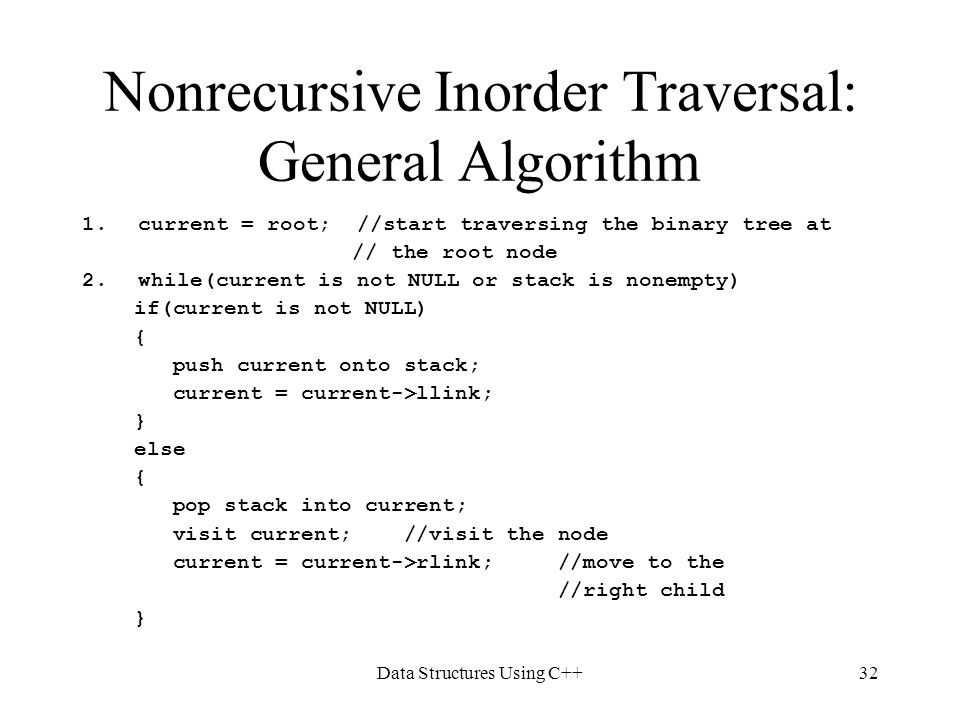# C Program – Inorder Tree Traversal without Recursioninorder tree travesal without recursion - learn in 30 secfrom microsoft awarded MVP,Using Stack is the obvious way to traverse tree without recursion. Below is an algorithm for traversing binary tree using stack. See this for step wise step execution of the algorithm.

Using Stack is the obvious way to traverse tree without recursion. Below is an algorithm for traversing binary tree using stack. See this for step wise step execution of the algorithm.

```1) Create an empty stack S.
2) Initialize current node as root
3) Push the current node to S and set current = current->left until current is NULL
4) If current is NULL and stack is not empty then
a) Pop the top item from stack.
b) Print the popped item, set current = popped_item->right
c) Go to step 3.
5) If current is NULL and stack is empty then we are done.
```

Let us consider the below tree for example

```            1
/   \
2      3
/  \
4     5

Step 1 Creates an empty stack: S = NULL

Step 2 sets current as address of root: current -> 1

Step 3 Pushes the current node and set current = current->left until current is NULL
current -> 1
push 1: Stack S -> 1
current -> 2
push 2: Stack S -> 2, 1
current -> 4
push 4: Stack S -> 4, 2, 1
current = NULL

Step 4 pops from S
a) Pop 4: Stack S -> 2, 1
b) print "4"
c) current = NULL /*right of 4 */ and go to step 3
Since current is NULL step 3 doesn't do anything.

Step 4 pops again.
a) Pop 2: Stack S -> 1
b) print "2"
c) current -> 5/*right of 2 */ and go to step 3

Step 3 pushes 5 to stack and makes current NULL
Stack S -> 5, 1
current = NULL

Step 4 pops from S
a) Pop 5: Stack S -> 1
b) print "5"
c) current = NULL /*right of 5 */ and go to step 3
Since current is NULL step 3 doesn't do anything

Step 4 pops again.
a) Pop 1: Stack S -> NULL
b) print "1"
c) current -> 3 /*right of 5 */

Step 3 pushes 3 to stack and makes current NULL
Stack S -> 3
current = NULL

Step 4 pops from S
a) Pop 3: Stack S -> NULL
b) print "3"
c) current = NULL /*right of 3 */```
``````#include<stdio.h>
#include<stdlib.h>
#define bool int

/* A binary tree tNode has data, pointer to left child
and a pointer to right child */
struct tNode
{
int data;
struct tNode* left;
struct tNode* right;
};

/* Structure of a stack node. Linked List implementation is used for
stack. A stack node contains a pointer to tree node and a pointer to
next stack node */
struct sNode
{
struct tNode *t;
struct sNode *next;
};

/* Stack related functions */
void push(struct sNode** top_ref, struct tNode *t);
struct tNode *pop(struct sNode** top_ref);
bool isEmpty(struct sNode *top);

/* Iterative function for inorder tree traversal */
void inOrder(struct tNode *root)
{
/* set current to root of binary tree */
struct tNode *current = root;
struct sNode *s = NULL;  /* Initialize stack s */
bool done = 0;

while (!done)
{
/* Reach the left most tNode of the current tNode */
if(current !=  NULL)
{
/* place pointer to a tree node on the stack before traversing
the node's left subtree */
push(&s, current);
current = current->left;
}

/* backtrack from the empty subtree and visit the tNode
at the top of the stack; however, if the stack is empty,
you are done */
else
{
if (!isEmpty(s))
{
current = pop(&s);
printf("%d ", current->data);

/* we have visited the node and its left subtree.
Now, it's right subtree's turn */
current = current->right;
}
else
done = 1;
}
} /* end of while */
}

/* UTILITY FUNCTIONS */
/* Function to push an item to sNode*/
void push(struct sNode** top_ref, struct tNode *t)
{
/* allocate tNode */
struct sNode* new_tNode =
(struct sNode*) malloc(sizeof(struct sNode));

if(new_tNode == NULL)
{
printf("Stack Overflow \n");
getchar();
exit(0);
}

/* put in the data  */
new_tNode->t  = t;

/* link the old list off the new tNode */
new_tNode->next = (*top_ref);

/* move the head to point to the new tNode */
(*top_ref)    = new_tNode;
}

/* The function returns true if stack is empty, otherwise false */
bool isEmpty(struct sNode *top)
{
return (top == NULL)? 1 : 0;
}

/* Function to pop an item from stack*/
struct tNode *pop(struct sNode** top_ref)
{
struct tNode *res;
struct sNode *top;

/*If sNode is empty then error */
if(isEmpty(*top_ref))
{
printf("Stack Underflow \n");
getchar();
exit(0);
}
else
{
top = *top_ref;
res = top->t;
*top_ref = top->next;
free(top);
return res;
}
}

/* Helper function that allocates a new tNode with the
given data and NULL left and right pointers. */
struct tNode* newtNode(int data)
{
struct tNode* tNode = (struct tNode*)
malloc(sizeof(struct tNode));
tNode->data = data;
tNode->left = NULL;
tNode->right = NULL;

return(tNode);
}

/* Driver program to test above functions*/
int main()
{

/* Constructed binary tree is
1
/   \
2      3
/  \
4     5
*/
struct tNode *root = newtNode(1);
root->left        = newtNode(2);
root->right       = newtNode(3);
root->left->left  = newtNode(4);
root->left->right = newtNode(5);

inOrder(root);

getchar();
return 0;
}``````

Time Complexity: O(n)

READ  Java Algorithm - Iterative Postorder Traversal | Set 2 (Using One Stack)

Output:

` 4 2 5 1 3`#### Venkatesan Prabu

Wikitechy Founder, Author, International Speaker, and Job Consultant. My role as the CEO of Wikitechy, I help businesses build their next generation digital platforms and help with their product innovation and growth strategy. I'm a frequent speaker at tech conferences and events.

X